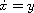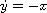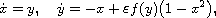Electron. J. Diff. Equ., Vol. 2014 (2014), No. 120, pp. 1-22.

### Generalized Van der Pol equation and Hilbert's 16th problem Xenakis Ioakim

Abstract:
In this article, we study the bifurcation of limit cycles from the harmonic oscillator,in the systemwhereis a small positive parameter tending to 0 and f is an odd polynomial of degree 2n + 1, with n an arbitrary but fixed natural number. We prove that, the above differential system, in the global plane, for particularly chosen odd polynomials f of degree 2n + 1 has exactly n + 1 limit cycles and that this number is an upper bound for the number of limit cycles for every case of an arbitrary odd polynomial f of degree 2n + 1. More specifically, the existence of the limit cycles, which is the first of the main results in this work, is obtained by using the Poincare's method, and the upper bound for the number of limit cycles can be derived from the work of Iliev . We also investigate the possible relative positions of the limit cycles for this differential system, which is the second main problem studying in this work. In particular, we construct differential systems with n given limit cycles and one limit cycle whose position depends on the position of the previous n limit cycles. Finally, we give some examples in order to illustrate the general theory presented in this work.

Submitted September 23, 2013. Published May 2, 2014.
Math Subject Classifications: 34C07, 34C23, 34C25.
Key Words: Generalized Van der Pol equation; Hilbert's 16th problem; limit cycle; existence; sinusoidal-type number; sinusoidal-type set; dependent radius; lambda-point.

Show me the PDF file (304 KB), TEX file, and other files for this article.Xenakis Ioakim Department of Mathematics and Statistics University of Cyprus P.O. Box 20537, 1678 Nicosia, Cyprus email: xioaki01@ucy.ac.cy